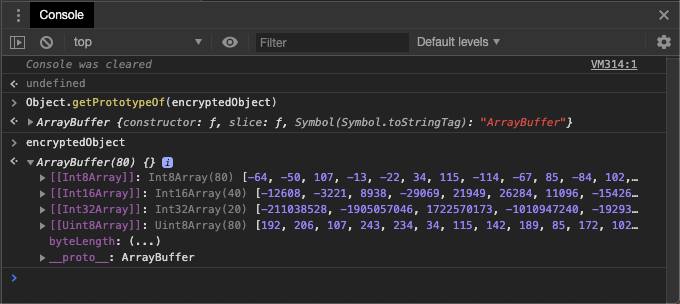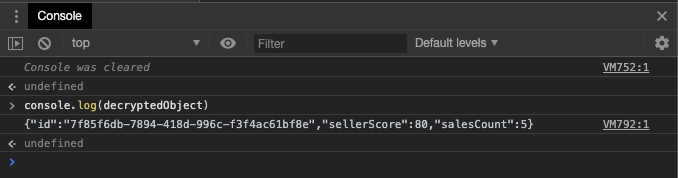White Paper on The New Generation of Invisible Bot Attacks. Download Now.
Back to posts

# Fun Times With WebCrypto — Part 2: Encrypting & Decrypting

May 28, 2019
•• Johnny Tordgeman
• Software EngineerJason Blackeye

With the PBKDF2 derivation in hand (if you need a refresher, check out part 1), its time to do something useful with WebCrypto and actually encrypt and decrypt an object.

## A Short Introduction to Encryption

In this post, we are going to use AES 256 CBC. I realized that although it is a very widely used encryption algorithm, many people using it know very little about it, so I decided to at least address a few basic terms.

What is AES?
AES stands for Advanced Encryption Standard and is a symmetric block encryption algorithm. Symmetric encryption means that the key used for encryption and decryption is identical, as opposed to asymmetric encryption where there are two keys; a private key and a public key. The public key is used to encrypt data, and only someone with the private key can read the encrypted data. The block size of AES is 128 bits. This means that the algorithms work in chunks of 128 bits.

When is AES Used?
AES is widely used in secure protocols such as HTTPS, FTPS and many more. It is very common for modern secure transfer protocols to use both symmetric and asymmetric encryption algorithms for different stages of communication. In the first step, the two parties use an asymmetric encryption algorithm to authenticate and agree on a symmetric key to use later when transferring the data. For example, the cypher suite TLS_DH_WITH_AES_256_CBC_SHA256 is composed of:

• Protocol name: TLS.
• Key exchange: TLS-DH is the key exchange method that uses the Diffie-Hellman algorithm.
• Encryption algorithm: AES_256_CBC.
• Integrity algorithm: SHA256.

## Let’s Encrypt!

Before we run ahead and start encrypting stuff, let’s define the how and the what of our encryption task.

We are going to encrypt a JSON object containing some data about a random user:

As we specified in Part 1, the WebCrypto module is part of a bigger system with the following requirements:

• Use PBKDF2 to reduce the vulnerability of our encryption key
• The encryption algorithm will be AES-CBC with a key size of 256-bit.

With this information in hand, we can get started with our first task: generating the encryption key.

## Generating The CryptoKey Object

When heading out to encrypt an object, we need to differentiate between two common scenarios:

• We were provided with a password for the key.
• It’s the first time we are encrypting this object and we need to generate a key for the encryption process.

👉 Generating a new key is done using the generateKey method of the crypto’s subtle object and will not be covered in this post.

The key (pun intended) to importing an existing key is to use theimportKey method of the crypto’s subtle object. This method requires the following parameters:

• Format: Defines the format of the key that is about to be imported. Possible values are: raw , pkcs8, spki or jwk .
• KeyData: The content of the key as either anarrayBuffer or JWK .
• Algorithm: An object describing the algorithm used for the key.
• Extractability: - A boolean parameter indicating whether the key should be extractable or not (i.e can it be exported for later use). Usages - An array indicating what will be the key usages (for example encrypting or decrypting items).

As our requirements state, we need to use the PBKDF2 hashing algorithm. In part 1 we created the first step for this - the derivation:

With the derivation buffer array in hand, we need to derive the key and the IV (initialization vector). To do this, we first need to know the length of each object. I used the hash algorithm SHA-256, which has a native size of 32 bytes. For the IV, I’ll set the size to 16 bytes. With these sizes, we can go ahead and slice the derivation array to a derivedKey array and an IV array:

Using the derivedKey arrayBuffer, we can import the key using the importKey method. Once we have the CryptoKey object in hand, let’s return it together with the IV array:

## Encrypting Stuff

With the key and IV in hand, its time to encrypt some stuff!

via GIPHY

Encrypting is done using the encrypt method of the crypto’s subtle object, which requires the following parameters:

• Algorithm: An object describing the encryption’s function algorithm.
• Key: ACryptoKey object containing the key used for the encryption.
• Data: An arrayBuffer containing the data to be encrypted.

We now have everything we need to encrypt the text. Let’s call the encrypt method with the following parameters:

If you check the type of encryptedObject using Object.getPrototypeOf you’ll see that it is an ArrayBuffer, which is exactly what the decrypt’s method expects.## Decrypting Stuff

Now that we have an encrypted object, let’s decrypt it and get it back to its true form!In order to decrypt an object, we need to use the same key that was used to encrypt it. In addition to that, if the key was hashed in a certain way (i.e pbkdf2), we would also need all of the information for that as well. In the previous section, we created 2 functions just for that: getDerivation and getKey which handles all of the aspects of importing a cryptoKey object.

Decrypting is done using the decrypt method of the crypto’ssubtle and it requires the exact same params as the encrypt function mentioned above.

Since we already have all the data we need from our encryption method, all we have to do now is pass the encrypted object (in bufferArray format) to the decryption method and, using TextDecoder, decode the result back to text:

Calling the new decrypt method will result as follows:## To sum it all up!

With the ability to encrypt and decrypt we can now leverage WebCrypto for some of our crypto needs, to improve security and boost client-side performance in case we wish to store secure data on the client. As always, there’s room for improvement with this code, but I believe it’s a pretty good way to get started with WebCrypto’s awesome encoding and decoding abilities.

While the process might seem long and tedious it’s actually a lot easier than you think. You can find a complete example https://github.com/pxjohnny/WebCrypto2Example

Let me know if you have any suggestions or other use cases for WebCrypto. The comments section is waiting :)